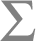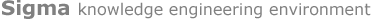Source: http://sigma2.cim3.net:8080/sigma/Browse.jsp?lang=en&kb=SUMO&term=TimePointBrowsing Interface [ Home |  Ask/Tell |  Graph |  Prefs ]KB:  SUMO Language:  en

 KB Term:English Word:Noun Verb Adjective Adverb

TimePoint(time point)

appearance as argument number 1(documentation TimePoint "An extensionless point on the universal timeline. The TimePoints at which Processes occur can be known with various degrees of precision and approximation, but conceptually TimePoints are point-like and not interval-like. That is, it doesn't make sense to talk about how long a TimePoint lasts.") Merge.kif 1664-1668 (subclass TimePoint TimePosition) Merge.kif 1663-1663 time point is a subclass of time position

appearance as argument number 2(instance NegativeInfinity TimePoint) Merge.kif 5893-5893 NegativeInfinity is an instance of time point (instance PositiveInfinity TimePoint) Merge.kif 5876-5876 PositiveInfinity is an instance of time point (range BeginFn TimePoint) Merge.kif 5983-5983 range of BeginFn is an instance of time point (range EndFn TimePoint) Merge.kif 6004-6004 range of EndFn is an instance of time point (termFormat en TimePoint "time point") english_format.kif 557-557 termFormat en time point "time point"

appearance as argument number 3(domain TimeIntervalFn 1 TimePoint) Merge.kif 6254-6254 the number 1 argument of TimeIntervalFn is an instance of time point (domain TimeIntervalFn 2 TimePoint) Merge.kif 6255-6255 the number 2 argument of TimeIntervalFn is an instance of time point (domain WhereFn 2 TimePoint) Merge.kif 3235-3235 the number 2 argument of WhereFn is an instance of time point (domain before 1 TimePoint) Merge.kif 6069-6069 the number 1 argument of before is an instance of time point (domain before 2 TimePoint) Merge.kif 6070-6070 the number 2 argument of before is an instance of time point (domain beforeOrEqual 1 TimePoint) Merge.kif 6106-6106 the number 1 argument of beforeOrEqual is an instance of time point (domain beforeOrEqual 2 TimePoint) Merge.kif 6107-6107 the number 2 argument of beforeOrEqual is an instance of time point (domain temporallyBetween 1 TimePoint) Merge.kif 6120-6120 the number 1 argument of temporallyBetween is an instance of time point (domain temporallyBetween 2 TimePoint) Merge.kif 6121-6121 the number 2 argument of temporallyBetween is an instance of time point (domain temporallyBetween 3 TimePoint) Merge.kif 6122-6122 the number 3 argument of temporallyBetween is an instance of time point (domain temporallyBetweenOrEqual 1 TimePoint) Merge.kif 6136-6136 the number 1 argument of temporallyBetweenOrEqual is an instance of time point (domain temporallyBetweenOrEqual 2 TimePoint) Merge.kif 6137-6137 the number 2 argument of temporallyBetweenOrEqual is an instance of time point (domain temporallyBetweenOrEqual 3 TimePoint) Merge.kif 6138-6138 the number 3 argument of temporallyBetweenOrEqual is an instance of time point (partition TimePosition TimeInterval TimePoint) Merge.kif 1652-1652 time position is exhaustively partitioned into time point

antecedent(<=>     (and         (time ?PHYS ?TIME)         (instance ?TIME TimePoint))     (temporallyBetweenOrEqual         (BeginFn             (WhenFn ?PHYS)) ?TIME         (EndFn             (WhenFn ?PHYS)))) Merge.kif 6150-6154 ?PHYS exists during ?TIME and ?TIME is an instance of time point if and only if ?TIME is between or at the beginning of the time of existence of ?PHYS and the end of the time of existence of ?PHYS (=>     (and         (instance ?POINT TimePoint)         (not             (equal ?POINT NegativeInfinity)))     (before NegativeInfinity ?POINT)) Merge.kif 5897-5901 if ?POINT is an instance of time point and ?POINT is not equal to NegativeInfinity, then NegativeInfinity happen%%{s} before ?POINT (=>     (and         (instance ?POINT TimePoint)         (not             (equal ?POINT NegativeInfinity)))     (exists (?OTHERPOINT)         (temporallyBetween NegativeInfinity ?OTHERPOINT ?POINT))) Merge.kif 5903-5908 if ?POINT is an instance of time point and ?POINT is not equal to NegativeInfinity, then there exists ?OTHERPOINT so that ?OTHERPOINT is between NegativeInfinity and ?POINT (=>     (and         (instance ?POINT TimePoint)         (not             (equal ?POINT PositiveInfinity)))     (before ?POINT PositiveInfinity)) Merge.kif 5880-5884 if ?POINT is an instance of time point and ?POINT is not equal to PositiveInfinity, then ?POINT happen%%{s} before PositiveInfinity (=>     (and         (instance ?POINT TimePoint)         (not             (equal ?POINT PositiveInfinity)))     (exists (?OTHERPOINT)         (temporallyBetween ?POINT ?OTHERPOINT PositiveInfinity))) Merge.kif 5886-5891 if ?POINT is an instance of time point and ?POINT is not equal to PositiveInfinity, then there exists ?OTHERPOINT so that ?OTHERPOINT is between ?POINT and PositiveInfinity (=>     (and         (instance ?POINT1 TimePoint)         (instance ?POINT2 TimePoint)         (instance ?INTERVAL TimeInterval)         (equal             (TimeIntervalFn ?POINT1 ?POINT2) ?INTERVAL))     (and         (equal             (BeginFn ?INTERVAL) ?POINT1)         (equal             (EndFn ?INTERVAL) ?POINT2))) Merge.kif 6261-6269 if ?POINT1 is an instance of time point and ?POINT2 is an instance of time point and ?INTERVAL is an instance of time interval and interval between ?POINT1 and ?POINT2 is equal to ?INTERVAL, then the beginning of ?INTERVAL is equal to ?POINT1 and the end of ?INTERVAL is equal to ?POINT2 (=>     (and         (instance ?POINT1 TimePoint)         (instance ?POINT2 TimePoint)         (instance ?INTERVAL TimeInterval)         (equal             (TimeIntervalFn ?POINT1 ?POINT2) ?INTERVAL))     (forall (?POINT)         (<=>             (temporallyBetweenOrEqual ?POINT1 ?POINT ?POINT2)             (temporalPart ?POINT ?INTERVAL)))) Merge.kif 6271-6280 if ?POINT1 is an instance of time point and ?POINT2 is an instance of time point and ?INTERVAL is an instance of time interval and interval between ?POINT1 and ?POINT2 is equal to ?INTERVAL, then for all ?POINT holds: ?POINT is between or at ?POINT1 and ?POINT2 if and only if ?POINT is a part of ?INTERVAL (=>     (and         (instance ?TIME TimePoint)         (holdsDuring ?TIME             (age ?OBJ ?DURATION)))     (duration         (TimeIntervalFn             (BeginFn                 (WhenFn ?OBJ)) ?TIME) ?DURATION)) Merge.kif 5724-5728 if ?TIME is an instance of time point and the age of ?OBJ is ?DURATION holds during ?TIME, then duration of interval between the beginning of the time of existence of ?OBJ and ?TIME is ?DURATION (=>     (instance ?POINT TimePoint)     (exists (?INTERVAL)         (and             (instance ?INTERVAL TimeInterval)             (temporalPart ?POINT ?INTERVAL)))) Merge.kif 5946-5951 if ?POINT is an instance of time point, then there exists ?INTERVAL so that ?INTERVAL is an instance of time interval and ?POINT is a part of ?INTERVAL

consequent(=>     (equal         (TemporalCompositionFn ?INTERVAL ?INTERVAL-TYPE) ?CLASS)     (forall (?TIME)         (=>             (and                 (instance ?TIME TimePoint)                 (temporalPart ?TIME ?INTERVAL))             (exists (?INSTANCE)                 (and                     (instance ?INSTANCE ?CLASS)                     (temporalPart ?TIME ?INSTANCE)))))) Merge.kif 6916-6926 if decomposition of ?INTERVAL into TemporalCompositionFn ?INTERVAL-TYPEs is equal to ?CLASS, then for all ?TIME holds: if ?TIME is an instance of time point and ?TIME is a part of ?INTERVAL, then there exists ?INSTANCE so that ?INSTANCE is an instance of ?CLASS and ?TIME is a part of ?INSTANCE (=>     (instance ?INTERVAL TimeInterval)     (exists (?POINT)         (and             (instance ?POINT TimePoint)             (temporalPart ?POINT ?INTERVAL)))) Merge.kif 5953-5958 if ?INTERVAL is an instance of time interval, then there exists ?POINT so that ?POINT is an instance of time point and ?POINT is a part of ?INTERVAL (=>     (instance ?OBJ Object)     (exists (?TIME1 ?TIME2)         (and             (instance ?TIME1 TimePoint)             (instance ?TIME2 TimePoint)             (before ?TIME1 ?TIME2)             (forall (?TIME)                 (=>                     (and                         (beforeOrEqual ?TIME1 ?TIME)                         (beforeOrEqual ?TIME ?TIME2))                     (time ?OBJ ?TIME)))))) Merge.kif 6077-6089 if ?OBJ is an instance of object, then there exist ?TIME1 and ?TIME2 so that ?TIME1 is an instance of time point and ?TIME2 is an instance of time point and ?TIME1 happen%%{s} before ?TIME2 and for all ?TIME holds: if ?TIME1 happen%%{s} before or at ?TIME and ?TIME happen%%{s} before or at ?TIME2, then ?OBJ exists during ?TIME